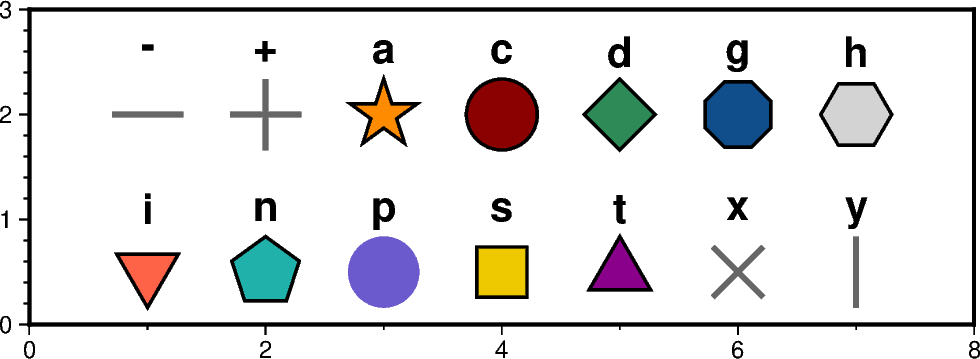# Basic geometric symbols

The `pygmt.Figure.plot` method can plot individual geometric symbols by passing the corresponding shortcuts to the `style` parameter. The 14 basic geometric symbols are shown underneath their corresponding shortcut codes. Four symbols (-, +, x and y) are line-symbols only for which we can adjust the linewidth via the `pen` parameter. The point symbol (p) only takes a color fill which we can define via the `fill` parameter. For the remaining symbols we may define a linewidth as well as a color fill.```import pygmt

fig = pygmt.Figure()
fig.basemap(region=[0, 8, 0, 3], projection="X12c/4c", frame=True)

# define fontstlye for annotations
font = "15p,Helvetica-Bold"

# upper row
y = 2

# use a dash in x direction (-) with a size of 0.9 cm,
# linewidth is set to 2p and the linecolor to "gray40"
fig.plot(x=1, y=y, style="-0.9c", pen="2p,gray40")
fig.text(x=1, y=y + 0.6, text="-", font=font)

# use a plus (+) with a size of 0.9 cm,
# linewidth is set to 2p and the linecolor to "gray40"
fig.plot(x=2, y=y, style="+0.9c", pen="2p,gray40")
fig.text(x=2, y=y + 0.6, text="+", font=font)

# use a star (a) with a size of 0.9 cm,
# linewidth is set to 1p, the linecolor to "black" (default) and the
# color fill to "darkorange"
fig.plot(x=3, y=y, style="a0.9c", pen="1p,black", fill="darkorange")
fig.text(x=3, y=y + 0.6, text="a", font=font)

# use a circle (c) with a size of 0.9 cm,
# linewidth is set to 1p, the linecolor to "black" and the
# color fill to "darkred"
fig.plot(x=4, y=y, style="c0.9c", pen="1p,black", fill="darkred")
fig.text(x=4, y=y + 0.6, text="c", font=font)

# use a diamond (d) with a size of 0.9 cm,
# linewidth is set to 1p, the linecolor to "black" and the
# color fill to "seagreen"
fig.plot(x=5, y=y, style="d0.9c", pen="1p,black", fill="seagreen")
fig.text(x=5, y=y + 0.6, text="d", font=font)

# use a octagon (g) with a size of 0.9 cm,
# linewidth is set to 1p, the linecolor to "black" and the
# color fill to "dodgerblue4"
fig.plot(x=6, y=y, style="g0.9c", pen="1p,black", fill="dodgerblue4")
fig.text(x=6, y=y + 0.6, text="g", font=font)

# use a hexagon (h) with a size of 0.9 cm,
# linewidth is set to 1p, the linecolor to "black" and the
# color fill to "lightgray"
fig.plot(x=7, y=y, style="h0.9c", pen="1p,black", fill="lightgray")
fig.text(x=7, y=y + 0.6, text="h", font=font)

# lower row
y = 0.5

# use an inverted triangle (i) with a size of 0.9 cm,
# linewidth is set to 1p, the linecolor to "black" and the
# color fill to "tomato"
fig.plot(x=1, y=y, style="i0.9c", pen="1p,black", fill="tomato")
fig.text(x=1, y=y + 0.6, text="i", font=font)

# use pentagon (n) with a size of 0.9 cm,
# linewidth is set to 1p, the linecolor to "black" and the
# color fill to "lightseagreen"
fig.plot(x=2, y=y, style="n0.9c", pen="1p,black", fill="lightseagreen")
fig.text(x=2, y=y + 0.6, text="n", font=font)

# use a point (p) with a size of 0.9 cm,
# color fill is set to "lightseagreen"
fig.plot(x=3, y=y, style="p0.9c", fill="slateblue")
fig.text(x=3, y=y + 0.6, text="p", font=font)

# use square (s) with a size of 0.9 cm,
# linewidth is set to 1p, the linecolor to "black" and the
# color fill to "gold2"
fig.plot(x=4, y=y, style="s0.9c", pen="1p,black", fill="gold2")
fig.text(x=4, y=y + 0.6, text="s", font=font)

# use triangle (t) with a size of 0.9 cm,
# linewidth is set to 1p, the linecolor to "black" and the
# color fill to "magenta4"
fig.plot(x=5, y=y, style="t0.9c", pen="1p,black", fill="magenta4")
fig.text(x=5, y=y + 0.6, text="t", font=font)

# use cross (x) with a size of 0.9 cm,
# linewidth is set to 2p and the linecolor to "gray40"
fig.plot(x=6, y=y, style="x0.9c", pen="2p,gray40")
fig.text(x=6, y=y + 0.6, text="x", font=font)

# use a dash in y direction (y) with a size of 0.9 cm,
# linewidth is set to 2p and the linecolor to "gray40"
fig.plot(x=7, y=y, style="y0.9c", pen="2p,gray40")
fig.text(x=7, y=y + 0.6, text="y", font=font)

fig.show()
```

Total running time of the script: (0 minutes 4.174 seconds)

Gallery generated by Sphinx-Gallery